﻿ (k,k-1)-双正则可图序列的公平划分 Judicious Balanced Bipartitions of (k,k-1)-Biregular Graphic Degree Sequence

Vol.07 No.04(2018), Article ID:24707,6 pages
10.12677/AAM.2018.74053

Judicious Balanced Bipartitions of (k, k − 1)-Biregular Graphic Degree Sequence

Haiyan Li, Jin Guo

College of Information Science and Technology, Hainan University, Haikou Hainan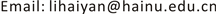Received: Apr. 11th, 2018; accepted: Apr. 21st, 2018; published: Apr. 28th, 2018ABSTRACT

Let $\pi =\left({d}_{1},{d}_{2},\cdots ,{d}_{n}\right)$ be a graphic sequence of nonnegative integers and ${\pi }_{1},{\pi }_{2}$ are two sequences that are obtained by partitioning the elements of π into two sets. A balanced bipartition of π is a bipartition ${\pi }_{1},{\pi }_{2}$ such that $-1\le |{\pi }_{1}|-|{\pi }_{2}|\le 1$ , where $|{\pi }_{i}|\left(i=1,2\right)$ is denoted to the number of elements of $|{\pi }_{i}|$ . In this paper, let k and m be positive integers, we determine the values ${\psi }_{max}\left(\pi \right)$ and ${\psi }_{min}\left(\pi \right)$ of $\left(k,k-1\right)$ -biregular graphic sequence $\pi =\left({k}^{m},{\left(k-1\right)}^{m}\right)$ .

Keywords:Graph, Degree Sequence, $\left({k}^{m},{\left(k-1\right)}^{m}\right)$ -Biregular Graphic Sequence, Judicious Balanced Bipartition(k, k − 1)-双正则可图序列的公平划分$\pi =\left({d}_{1},{d}_{2},\cdots ,{d}_{n}\right)$ 是非负整数序列， ${\pi }_{1},{\pi }_{2}$ 是将 $\pi$ 的所有元素划分为两部分后的两个子序列。如果 $-1\le |{\pi }_{1}|-|{\pi }_{2}|\le 1$ ，则称 ${\pi }_{1},{\pi }_{2}$$\pi$ 的一个平衡二部划分，其中 $|{\pi }_{i}|\left(i=1,2\right)$ 表示 ${\pi }_{i}$ 中的元素数目。设k和m是两个正整数， $\pi =\left({k}^{m},{\left(k-1\right)}^{m}\right)$ 是双正则可图序列。本文确定了 ${\psi }_{max}\left(\pi \right)$ 的值和 ${\psi }_{min}\left(\pi \right)$ 的值。1. 引言

${V}_{1},{V}_{2}$ 是图G的一个二部划分，如果 $-1\le |{V}_{1}|-|{V}_{2}|\le 1$ ，则称 ${V}_{1},{V}_{2}$ 是G的一个二部平衡划分。对于 $i=1,2$$e\left({V}_{i}\right)$ 表示两个端点都在 ${V}_{i}$ 中的边的数目， $e\left({V}_{1},{V}_{2}\right)$ 表示两个端点分别在顶点子集 ${V}_{1},{V}_{2}$ 中的边数。通常 $e\left({V}_{1},{V}_{2}\right)$ 用来表示平衡二部划分的大小。图G的一个最大(最小)平衡二部划分 ${V}_{1},{V}_{2}$ 是图G的所有平衡二部划分中 $e\left({V}_{1},{V}_{2}\right)$ 的值达到最大(最小)。与最大，最小平衡划分问题不同，公平划分问题是寻找图G的一个划分，使得多个分量同时进行优化。

2. 主要定理及引理

$\sum _{i=1}^{t}{d}_{i}\le t\left(t-1\right)+\sum _{j=t+1}^{n}\mathrm{min}\left\{{d}_{i},t\right\},\text{\hspace{0.17em}}1\le t\le n$

$P:=\left({p}_{1},{p}_{2},\cdots ,{p}_{m}\right)$$Q:=\left({q}_{1},{q}_{2},\cdots ,{q}_{n}\right)$ 是两个非负整数序列。如果存在一个简单二部图 $G\left[X,Y\right]$ 使得X和Y中的顶点度分别是 $\left({p}_{1},{p}_{2},\cdots ,{p}_{m}\right)$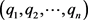，那么称序列对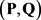是二部可图的，并称二部图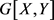的一个实现。Gale  和Ryser  分别独立地给出了关于二部可图序列的刻划定理。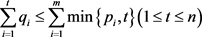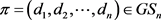，若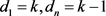，则称π是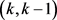-双正则可图的。本文主要给出双正则可图序列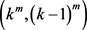的公平划分的上下界。

3. (k, k − 1)-双正则可图序列的公平划分的上界

1) 若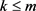，则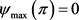2) 若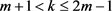，则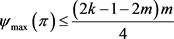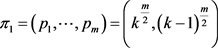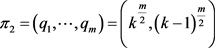，那么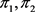是π的一个平衡二部(1)(2)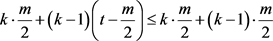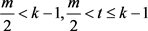，由(1)和(2)得，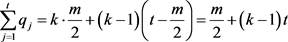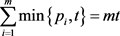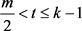可得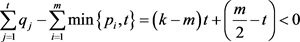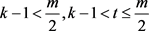，由(1)和(2)得，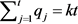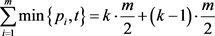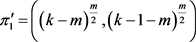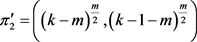。由于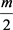是偶数，所以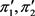的度和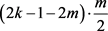为偶数。由引理2.3知，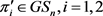。设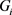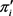的一个实现且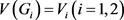。令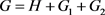。容易验证G是π的一个实现，且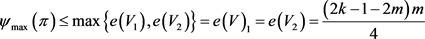4. (k, k-1)-双正则可图序列的公平划分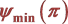的下界

1) 若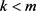，则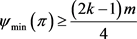2) 若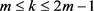，则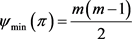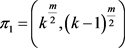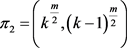，则是π的一个平衡二部划分。由于是偶数，所以的度和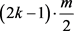为偶数。又由引理2.3可得，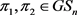。设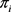的一个实现且，令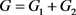。容易验证G是π的一个实现，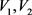是G的一个平衡二部划分且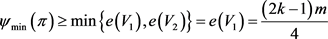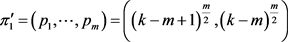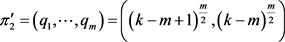。这里，(3)(4)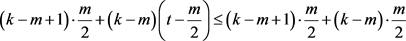，由(3)和(4)得，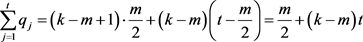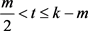可知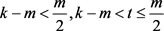，由(3)和(4)得，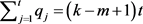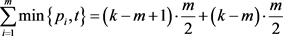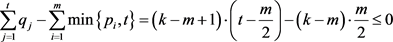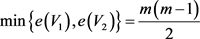Judicious Balanced Bipartitions of (k,k-1)-Biregular Graphic Degree Sequence[J]. 应用数学进展, 2018, 07(04): 423-428. https://doi.org/10.12677/AAM.2018.74053

1. 1. Bondy, J.A. and Murty, U.S.R. (1976) Graphy Theory with Applications. Macmillan Ltd Press, New York. https://doi.org/10.1007/978-1-349-03521-2

2. 2. Erdös, P. and Gallai, T. (1960) Graphs with Prescribed Degrees of Vertices. Matematikai Lapok, 11, 264-274.

3. 3. Gale, D. (1957) A Theorem on Flows in Networks. Pacific Journal of Mathematics, 7, 1073-1082. https://doi.org/10.2140/pjm.1957.7.1073

4. 4. Ryser, H.J. (1957) Combinatorial Properties of Matrices of Zeros and Ones. Canadian Journal of Mathematics, 9, 371-377. https://doi.org/10.4153/CJM-1957-044-3

5. 5. Yin, J.H. and Li, J.S. (2005) Two Sufficient Conditions for a Graphic Sequence to Have a Realization with Prescribed Clique Size. Discrete Mathematics, 301, 218-227. https://doi.org/10.1016/j.disc.2005.03.028